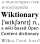Feeds:
Posts

# Computation

Computation is a general term for any type of information processing. This includes phenomena ranging from human thinking to calculations with a more narrow meaning. Computation is a process following a well-defined model that is understood and can be expressed in an algorithm, protocol, network topology, etc. Computation is also a major subject matter of computer science: it investigates what can or cannot be done in a computational manner.Look up computation in Wiktionary, the free dictionary.

[hide]

##  Classes of computation

Computation can be classified by at least three orthogonal criteria: digital vs analog, sequential vs parallel vs concurrent, batch vs interactive.

In practice, digital computation is often used to simulate natural processes (for example, Evolutionary computation), including those that are more naturally described by analog models of computation (for example, Artificial neural network). In this situation, it is important to distinguish between the mechanism of computation and the simulated model.

##  Computations as a physical phenomenon

A computation can be seen as a purely physical phenomenon occurring inside a closed physical system called a computer. Examples of such physical systems include digital computers, quantum computers, DNA computers, molecular computers, analog computers or wetware computers. This point of view is the one adopted by the branch of theoretical physics called the physics of computation.

An even more radical point of view is the postulate of digital physics that the evolution of the universe itself is a computation – Pancomputationalism.

##  Mathematical models of computation

In the theory of computation, a diversity of mathematical models of computers have been developed. Typical mathematical models of computers are the following:

• State models including Turing Machine, Push-down automaton, Finite state automaton, and PRAM
• Functional models including lambda calculus
• Logical models including logic programming
• Concurrent models including Actor model and process calculi

##  History

The word computation has an archaic meaning (from its Latin etymological roots), but the word has come back in use with the arising of a new scientific discipline: computer science.This computer science article is a stub. You can help Wikipedia by expanding it.